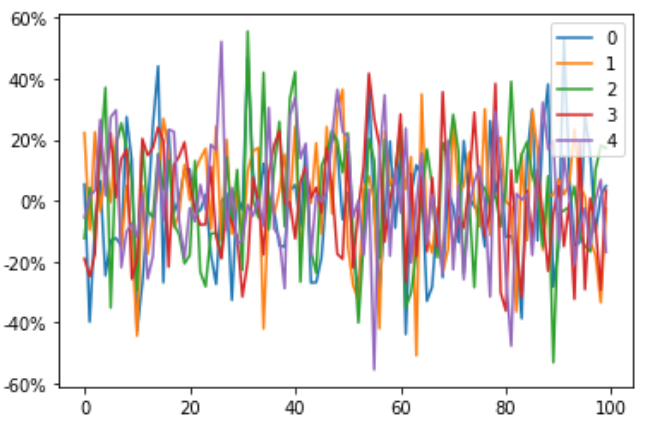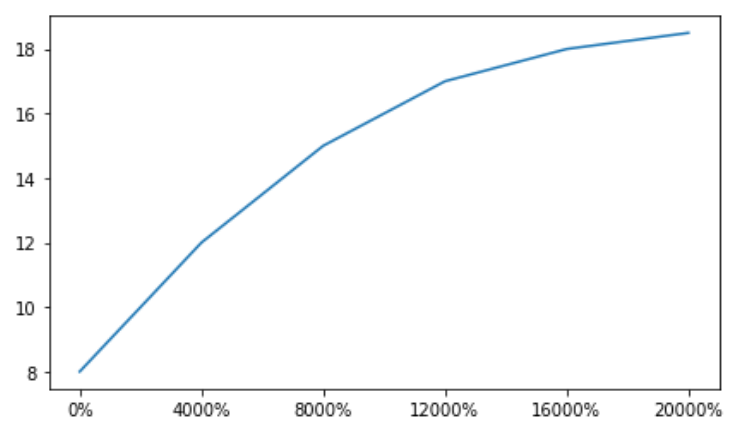# Matplotlib.ticker.PercentFormatter class in Python

Matplotlib is an amazing visualization library in Python for 2D plots of arrays. Matplotlib is a multi-platform data visualization library built on NumPy arrays and designed to work with the broader SciPy stack.

## matplotlib.ticker.PercentFormatter

The` matplotlib.ticker.PercentFormatter` class is used to format numbers as a percentage.

Syntax: class matplotlib.ticker.PercentFormatter(xmax=100, decimals=None, symbol=’%’, is_latex=False)

Parameters:

• xmax: It is a float value that determines how the number is converted into a percentage.
• decimals: It is either an integer value or None. It determines the number of decimal places to place after the point. If None (the default), the number will be computed automatically.
• symbol : It is either a string or none that gets appended to the label.
• is_latex: It is a boolean value. If False, reserved LaTeX characters in symbol are eliminated.

Example 1:

 `import` `pandas as pd ` `import` `numpy as np ` `import` `matplotlib.ticker as mtick ` `from` `matplotlib.ticker ``import` `PercentFormatter ` ` `  `df ``=` `pd.DataFrame(np.random.randn(``100``, ``5``)) ` ` `  `ax ``=` `df.plot() ` `ax.yaxis.set_major_formatter(mtick.PercentFormatter(``5.0``))  `

Output:Example 2:

 `import` `matplotlib.pyplot as plt ` `import` `numpy as np ` `import` `matplotlib.ticker as mtick ` `from` `matplotlib.ticker ``import` `PercentFormatter ` ` `  `data ``=` `[``8``, ``12``, ``15``, ``17``, ``18``, ``18.5``] ` `perc ``=` `np.linspace(``0``, ``100``, ``len``(data)) ` ` `  `fig ``=` `plt.figure(``1``, (``7``, ``4``)) ` `ax ``=` `fig.add_subplot(``1``, ``1``, ``1``) ` ` `  `ax.plot(perc, data) ` ` `  `xticks ``=` `mtick.PercentFormatter(``0.5``) ` `ax.xaxis.set_major_formatter(xticks) ` ` `  `plt.show() `

Output:My Personal Notes arrow_drop_upCheck out this Author's contributed articles.

If you like GeeksforGeeks and would like to contribute, you can also write an article using contribute.geeksforgeeks.org or mail your article to contribute@geeksforgeeks.org. See your article appearing on the GeeksforGeeks main page and help other Geeks.

Please Improve this article if you find anything incorrect by clicking on the "Improve Article" button below.

Article Tags :

Be the First to upvote.

Please write to us at contribute@geeksforgeeks.org to report any issue with the above content.